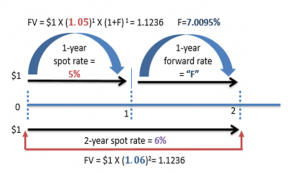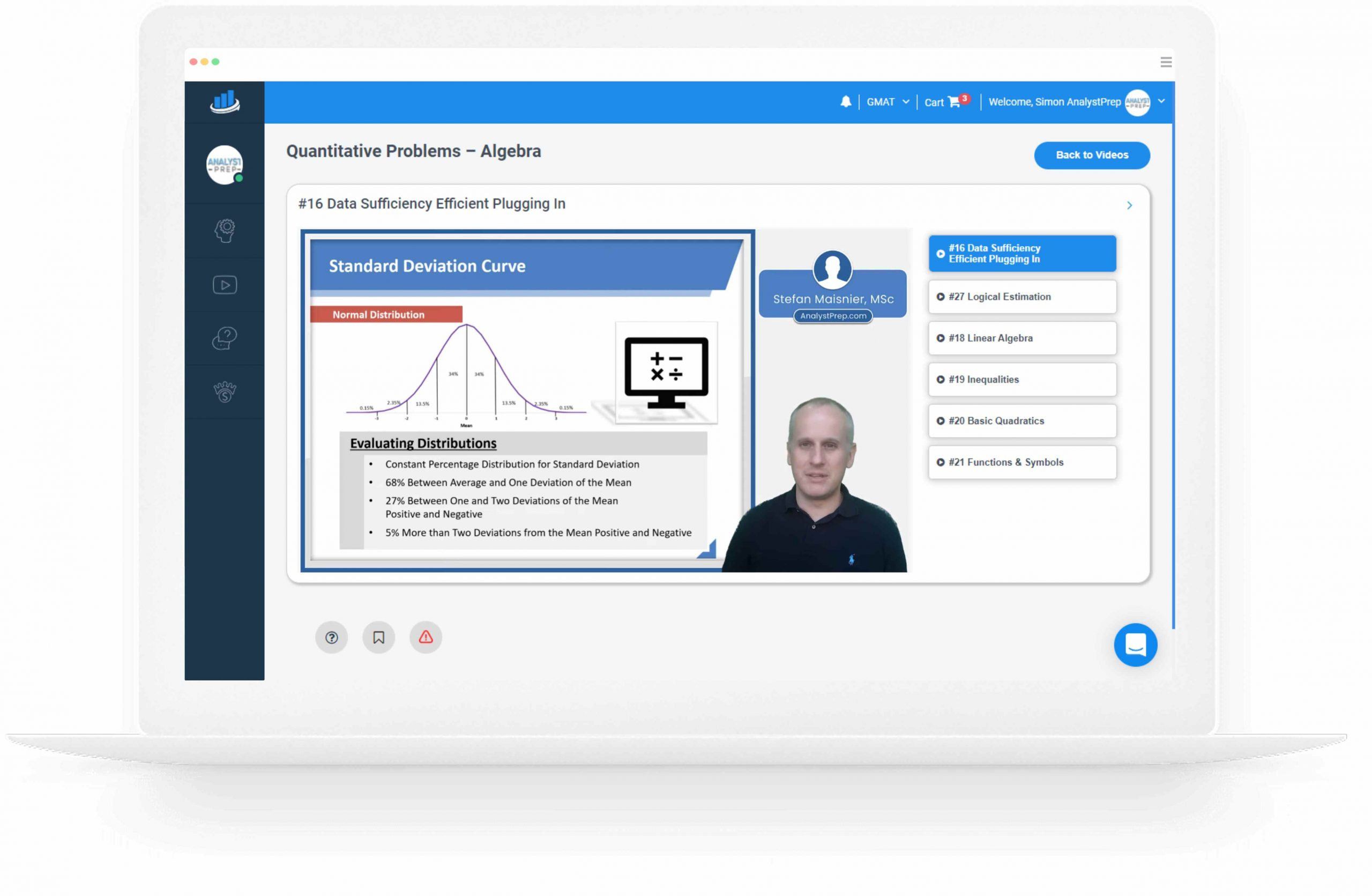# Spot Rate vs. Forward Rates (Calculations for CFA® and FRM® Exams)

## Spot Rates

A spot interest rate gives you the price of a financial contract on the spot date. The spot date is the day when the funds involved in a business transaction are transferred between the parties involved. It could be two days after a trade, or even on the same day the deal is completed. A spot rate of 5% is the agreed-upon market price of the transaction based on current buyer and seller action.

## Formula

The general formula for calculating a bond’s price given a sequence of spot rates is given below:

$${ PV }_{ bond }=\frac { PMT }{ { (1+{ Z }_{ 1 }) }^{ 1 } } +\frac { PMT }{ { (1+{ Z }_{ 2 }) }^{ 2 } } +…+\frac { PMT+Principal }{ { (1+{ Z }_{ n }) }^{ n } }$$

Where:

$${ PV }_{ bond }$$  is the present value of the price of the bond;

PMT is the coupon payment per period;

$${ Z }_{ 1 },{ Z }_{ 2 }$$ and $${ Z }_{ n }$$ are the spot rates for periods 1,2 and n respectively; and

n is the number of evenly spaced periods to maturity.

Suppose that:

• The 1-year spot rate is 3%;
• The 2-year spot rate is 4%; and
• The 3-year spot rate is 5%.

The price of a 100-par value 3-year bond paying 6% annual coupon payment is 102.95.

$$\begin{array}{l|cccccc} \text{Time Period} & 1 & 2 & 3 \\ \hline \text{Calculation} & \frac {\6}{{\left(1+3\%\right)}^{ 1 } } & \frac { \6 }{ { \left( 1+4\% \right) }^{ 2 } } & \frac { \106 }{ { \left( 1+5\% \right) }^{ 3 } } \\ \hline \text{Cash Flow} & \5.83 & +\5.55 & +\91.57 & =\102.95 \\ \end{array}$$

Spot rates are also applied in determining the yield to maturity of a bond.

## Calculating the Yield-to-maturity of a Bond using Spot Rates

Continuing on the same example, this 3-year bond is priced at a premium above par value, so its yield-to-maturity must be less than 6%. We can now use the financial calculator to find the yield-to-maturity using the following inputs:

• n = 3;
• PV = -102.95; (Since this is a cash outflow)
• PMT = 6; (Since this is a cash inflow for the investor)
• FV = 100; (Since this is a cash inflow for the investor)
• CPT => I/Y = 4.92 (Which signifies 4.92%)

The yield-to-maturity is found to be 4.92%, which we can confirm with the following calculation:

$$\begin{array}{l|cccccc} \text{Time Period} & 1 & 2 & 3 \\ \hline \text{Calculation} & \frac {\6}{{\left(1+4.92\%\right)}^{ 1 } } & \frac { \6 }{ { \left( 1+4.92\% \right) }^{ 2 } } & \frac { \106 }{ { \left( 1+4.92\% \right) }^{ 3 } } \\ \hline \text{Cash Flow} & \5.719 & +\5.450 & +\91.770 & =\102.95 \\ \end{array}$$

## Forward Rates

In theory, forward rates are prices of financial transactions that are expected to take place at some future point.

A forward rate indicates the interest rate on a loan beginning at some time in the future, whereas a spot rate is the interest rate on a loan beginning immediately. Thus, the forward market rate is for future delivery after the usual settlement time in the cash market.

## Deriving Forward Rates from a Set of Spot Rates

$${ Z }_{ n }$$, the $$n$$-year spot rate, is a measure of the average interest rate over the period from now until $$n$$ years’ time.

The forward rate, $${ f }_{ t,r }$$, is a measure of the average interest rate between times $$t$$ and $$t + r$$. It’s the interest rate agreed today $$(t=0)$$ on an investment made at time $$t>0$$ for a period of $$r$$ years.

The one-year forward rate, $${ f }_{ t,1 }$$, is therefore the rate of interest from time $$t$$ to time $$t +1$$. It can be expressed in terms of spot rates as follows:

$$1+{ f }_{ t,1 } = \frac{{{(1+{Z}_{t+1})}}^{t+1}}{{(1+{Z}_{t})}^{t}}$$

Alternatively,

Step 1: Use the formula:

$$1+{ f }_{ t,1 }=\frac {V_2}{V_1}$$

Where $$V_1$$ is the value to which a dollar grows by time $$T_1$$ and $$V_2$$ is the value to which a dollar grows by $$T_1$$.

Step 2: Calculate the interest rate that equates the value of one dollar at time $$T_1$$ to the value of one dollar at time $$T_2$$.

## Example: Calculating the one-year forward rate.

You are given the following spot rates:

• 1-year spot rate: 5%;
• 2-year spot rate: 6%.

Determine the one-year forward rate one year from today, i.e., $$f_{1,1}$$.

There are 2 ways to solve this question:

### 1. Using the Formula

$$1+{ f }_{ t,1 } = \frac{{{(1+{Z}_{t+1})}}^{t+1}}{{(1+{Z}_{t})}^{t}}$$

$$(1 + \text{1-year spot}) × (1 + \text{1-year forward rate at time 1}) = (1 + \text{2-year spot})^2$$

$$(1.05)\ × (1\ +\ f_{1,1}) = {(1.06)}^2$$

$$(f_{1,1}) = 7.0095%$$

###We use the principle of no-arbitrage. The golden rule to remember while using the timeline is that no matter which route you take to “time travel” from one period to the other- the end result (Future Value) will always be the same.

There are 2 possible routes we could take here

1. Invest for one year at the spot rate of 5% and then invest for another year at the unknown forward rate “F.”
2. Invest for two years at the spot rate of 6%.

As per the assumption of no-arbitrage, we should arrive at the same value for both.

By equating i & ii, we get F = 7%, which is the same as what we got using the formula.

FRM Part I & Part II Complete Course

Shop CFA® Exam Prep

Offered by AnalystPrepLevel I
Level II
Level III
All Three Levels
Featured Shop FRM® Exam PrepFRM Part I
FRM Part II
FRM Part I & Part II
Learn with Us

Subscribe to our newsletter and keep up with the latest and greatest tips for success

Shop Actuarial Exams PrepExam P (Probability)
Exam FM (Financial Mathematics)
Exams P & FM
Shop GMAT® Exam PrepComplete Course
Shop Executive Assessment® Exam PrepComplete Course